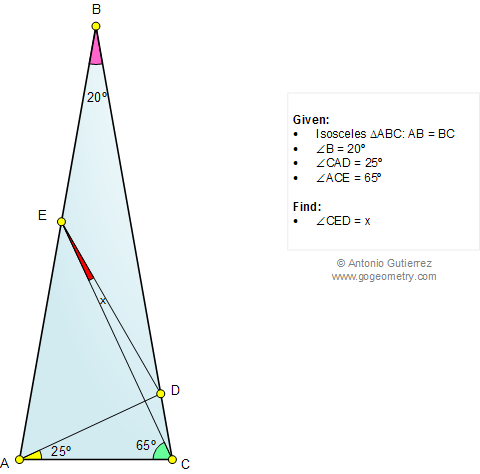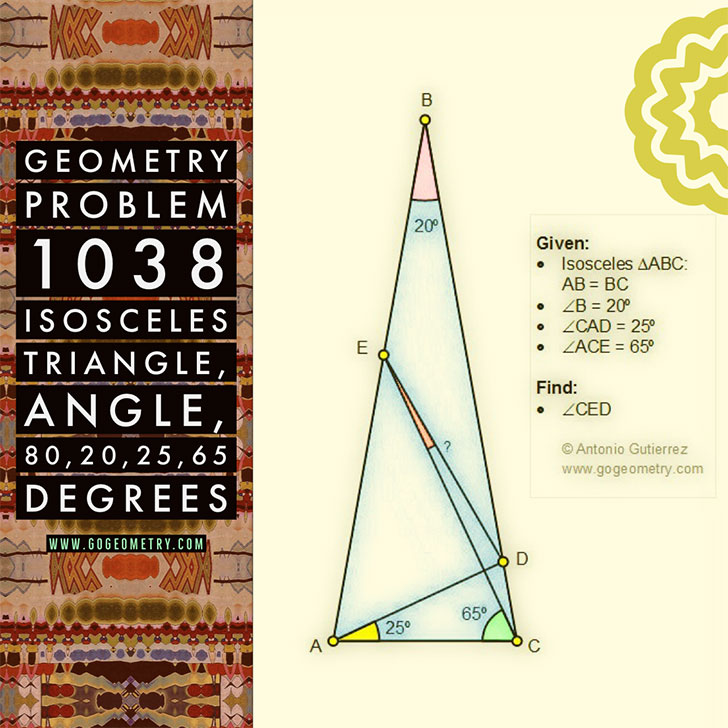# Online Math: Geometry Problem 1038: Isosceles Triangle, Angle, 80, 20, 25, 65 Degrees. Level: School, College.

< PREVIOUS PROBLEM  |  NEXT PROBLEM >

The figure below shows an isosceles triangle ABC with AB = BC, angle B = 20 degrees, D on BC and E on AB. If angle CAD = 25 degrees and angle ACE = 65 degrees, find the measure of angle CED.
An "elegant" solution must use only elementary geometry, no trigonometry.## Poster of: Geometry Problem 1038: Sketching, Typography using iPad Apps.